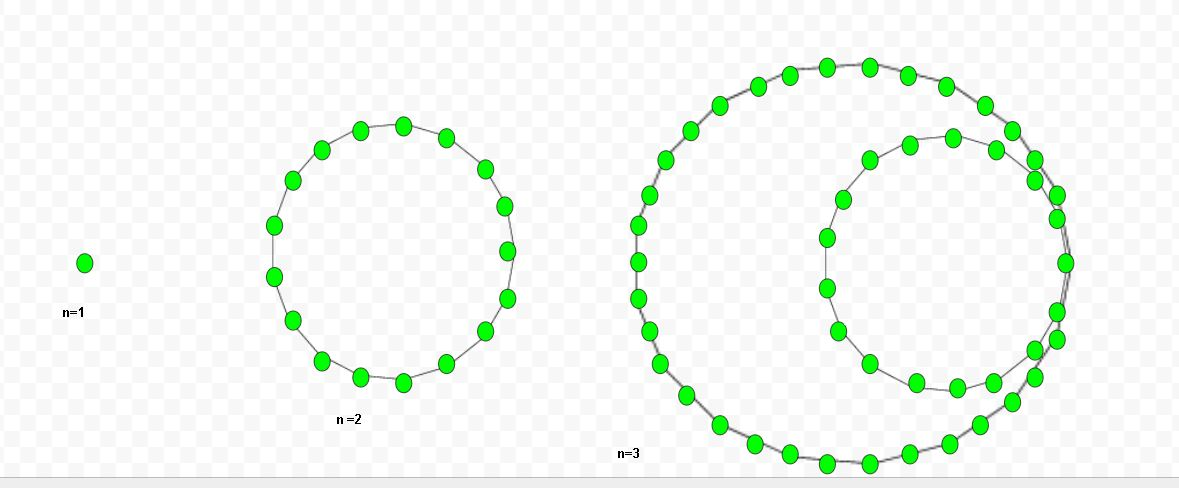• Last Updated : 19 May, 2022

Given a number n, the task is to find the nth heptadecagonal number .
A heptadecagonal number is a class of figurate numbers. It has a seventeen-sided polygon called heptadecagon. The n-th heptadecagonal number count’s the seventeen number of dots and all other dots are surrounding with a common sharing corner and make a pattern.

Examples:

Input : 5
Output :155
Input :9
Output :549Formula to calculate nth heptadecagonal number:## C++

 // C++ program to find Nth// heptadecagonal number#include using namespace std; // Function to calculate heptadecagonal// numberint heptadecagonalNum(long int n){    return ((15 * n * n) - 13 * n) / 2;} // Driver Codeint main(){    long int n = 3;    cout << n << "th Heptadecagonal number : ";    cout << heptadecagonalNum(n);    cout << endl;    n = 8;    cout << n << "th Heptadecagonal number : ";    cout << heptadecagonalNum(n);     return 0;}

## C

 // C program to find Nth// heptadecagonal number#include  // Function to calculate heptadecagonal// numberint heptadecagonalNum(long int n){    return ((15 * n * n) - 13 * n) / 2;} // Driver Codeint main(){    long int n = 3;    printf("%ldth Heptadecagonal number : ",n);    printf("%d\n",heptadecagonalNum(n));     n = 8;    printf("%ldth Heptadecagonal number : ",n);    printf("%d\n",heptadecagonalNum(n));     return 0;} // This code is contributed by kothavvsaakash

## Java

 // Java program to find Nth heptadecagonal numberimport java.io.*; class GFG {         // Function to calculate heptadecagonal    // number    static long heptadecagonalNum(long n)    {        return ((15 * n * n) - 13 * n) / 2;    }         // Driver Code    public static void main (String[] args)    {        long n = 3;        System.out.print( n + "th Heptadecagonal"                                 + " number : ");        System.out.println( heptadecagonalNum(n));                 n = 8;        System.out.print( n + "th Heptadecagonal"                                 + " number : ");        System.out.print( heptadecagonalNum(n));    }} // This code is contributed by anuj_67.

## Python3

 # Python program to find Nth# heptadecagonal number # Function to calculate# heptadecagonal numberdef heptadecagonalNum(n):     # Formula to calculate nth    # heptadecagonal number    return ((15 * n * n) - 13 * n) // 2  # Driver Coden = 3print("%sth Heptadecagonal number : " %n,                    heptadecagonalNum(n))n = 8print("%sth Heptadecagonal number: " %n,                    heptadecagonalNum(n))                                          # This code is contributed by ajit

## C#

 // C# program to find Nth// heptadecagonal numberusing System;class GFG {         // Function to calculate    // heptadecagonal number    static long heptadecagonalNum(long n)    {        return ((15 * n * n) -                  13 * n) / 2;    }         // Driver Code    public static void Main ()    {        long n = 3;        Console.Write( n + "th Heptadecagonal"                                + " number : ");        Console.WriteLine( heptadecagonalNum(n));                 n = 8;        Console.Write( n + "th Heptadecagonal"                                + " number : ");        Console.WriteLine( heptadecagonalNum(n));    }} // This code is contributed by anuj_67.

## PHP

 

## Javascript

 

Output

3th Heptadecagonal number : 48
8th Heptadecagonal number : 428

Time Complexity: O(1)
Auxiliary Space: O(1)

My Personal Notes arrow_drop_up